# Harmonic Forced Vibration of a Simply-supported Thin Square PlateThis test lets you check analysis results for a simply-supported thin square plate, in the context of an harmonic dynamic response case. You will use 2D meshes.

This test proposed by NAFEMS is used to validate the following attributes:

• 2D shell elements (i.e. the elementary stiffness and mass matrices)

• Harmonic dynamic response solve algorithms.Reference:

NAFEMS-Glasgow, Benchmark newsletter, Report No. E1261/R002, p.21, February 1989.

##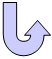Specifications

### Geometry Specifications

 Length: L = 10000 mm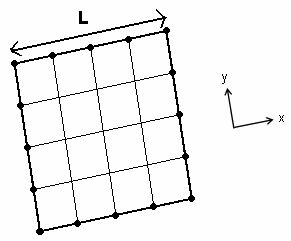Thickness: th = 50 mm

### Analysis Specifications

 Young Modulus (material) E = 200 GPa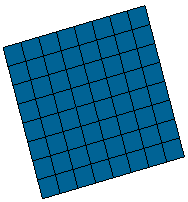Poisson's Ratio (material) ν = 0.3 Density: ρ = 8000 kg/m3 Restraints (User-defined): Tx = Ty = Rz = 0 at all nodes Tz = 0 along all four edges Rx = 0 along edges x = 0 and x = 10000 mm Ry = 0 along edges y = 0 and y = 10000 mm Loads: F = F0 sin wtwhere: F0 = 100 N/m2 w = 2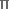f f = 0  to  4.16 Hz Damping d = 2% in all 16 modes used

##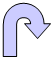Results

The results for different finite elements are presented in the table below.

The peak is the value at undamped natural frequency.
In this particular test, the undamped natural frequency is 2.377 Hz.

 Type of values Reference solution Values Linear triangle shell (TR3) 64 x 64 elements Parabolic triangle shell (TR6) 32 x 32 elements Linear quadrangle shell (QD4) 32 x 32 elements Parabolic quadrangle shell (QD8) 16 x 16 elements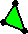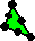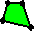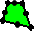Computed Results Error [%] Computed Results Error [%] Computed Results Error [%] Computed Results Error [%] Peak displacement [mm] at 2.377 Hz 45.420 45.430 0.023 45.430 0.022 45.477 0.125 45.429 0.020 Peak stress [MPa] 30.030 32.005 6.58 32.082 6.83 31.976 6.48 32.227 7.32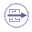To Perform the Test:

The Harmonic_forced_vibration_of_a_simply_supported_thin_square_plate.CATAnalysis document presents a complete analysis of this case, computed with a mesh formed of linear quadrangle elements (QD4).

To compute the case with parabolic quadrangle (QD8), linear triangle (TR3) and parabolic triangle (TR6) elements, proceed as follow:

1. Open the CATAnalysis document.

2. In the Advanced Meshing Tools workbench, replace the mesh specifications as indicated above.

3. In the Generative Structural Analysis workbench, compute the case.